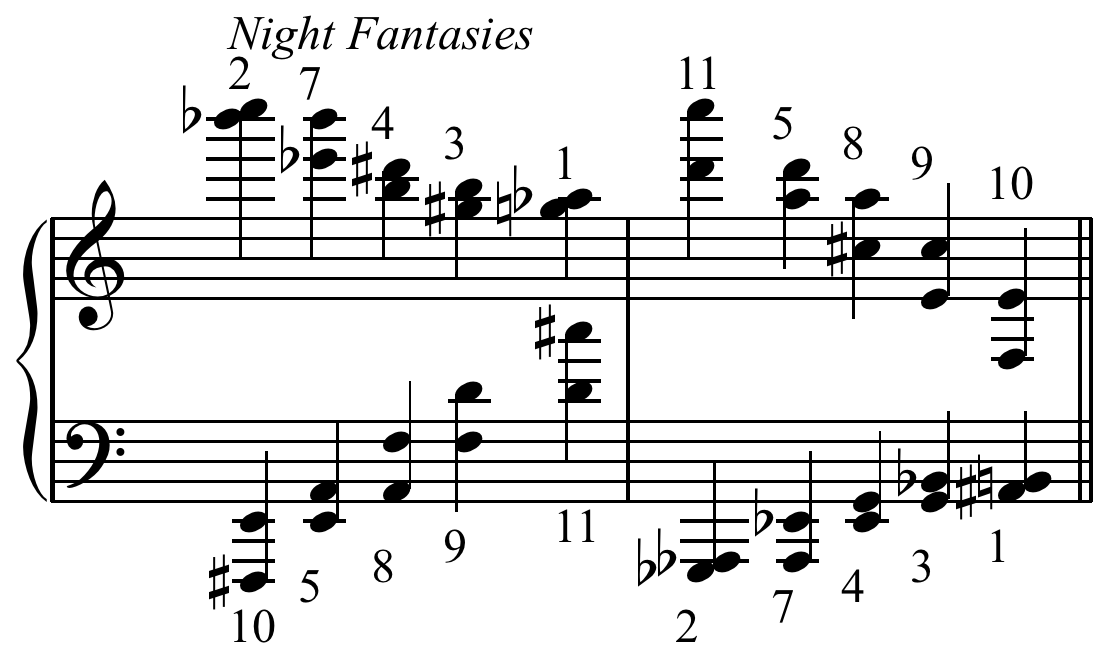PyCSP3

type: CSP
difficulty: easy

# Problem All-Interval Series

Given the twelve standard pitch-classes (c, c#, d, $\dots$), represented by numbers $0,1,\dots,11$, find a series in which each pitch-class occurs exactly once and in which the musical intervals between neighboring notes cover the full set of intervals from the minor second (1 semitone) to the major seventh (11 semitones). That is, for each of the intervals, there is a pair of neighboring pitch-classes in the series, between which this interval appears.

Elliott Carter often bases his all-interval sets on the list generated by Bauer-Mendelberg and Ferentz and uses them as a “tonic” sonority. Image from commons.wikimedia.orgThe problem of finding such a series can be easily formulated as an instance of a more general arithmetic problem. Given a positive integer $n$, find a sequence $x = \langle x_0, x_1, \dots, x_{n-1} \rangle$, such that:

• $x$ is a permutation of ${0,1,…,n-1}$;
• the interval sequence $y = \langle |x_1-x_0|, |x_2-x_1|, … |x_{n-1}-x_{n-2}| \rangle$ is a permutation of ${1,2,…,n-1}$.

A sequence satisfying these conditions is called an all-interval series of order $n$; the problem of finding such a series is the all-interval series problem of order $n$.

For example, for $n=8$, a solution is:

    1 7 0 5 4 2 6 3


To build a CSP (Constraint Satisfaction Problem) model, we need first to import the library PyCSP$^3$:

from pycsp3 import *


Then, we need some data. Actually, we just need an integer $n$. For example, the value 10.

n = 10


We start our CSP model by introducing an array $x$ of variables.

# x[i] is the ith note of the series
x = VarArray(size=n, dom=range(n))


We can display the structure of the array, as well as the domain of the first variable (note that all variables have the same domain).

print("Array x: ", x)
print("Domain of any variable: ", x.dom)

Array x:  [x, x, x, x, x, x, x, x, x, x]
Domain of any variable:  0..9


Concerning the constraints, we have to post two constraints AllDifferent: the first one on $x$, and the second one on expressions representing successive distances.

satisfy(
# notes must occur once, and so form a permutation
AllDifferent(x),

# intervals between neighbouring notes must form a permutation
AllDifferent(abs(x[i] - x[i + 1]) for i in range(n - 1))
);


We can display the internal representation of the two posted constraints; this way, although a little bit technical, we can see that the arguments of the two constraints are correct.

print(posted())

allDifferent(list:[x, x, x, x, x, x, x, x, x, x])
allDifferent(list:[dist(x,x), dist(x,x), dist(x,x), dist(x,x), dist(x,x), dist(x,x), dist(x,x), dist(x,x), dist(x,x)])


Interestingly, by calling the function solve(), we can check that the problem is satisfiable (SAT). We can also display the found solution. Here, we call the function values() that collects the values assigned to a specified list of variables.

if solve() is SAT:
print(values(x))

[4, 2, 5, 6, 0, 9, 1, 8, 3, 7]


One way of breaking some symmetries is to post this constraint:

satisfy(
# tag(symmetry-breaking)
x < x[n - 1]
);


Now, we can check that 148 solutions exist, as follows.

if solve(sols=ALL) is SAT:
print("Number of solutions: ", n_solutions())

Number of solutions:  148


Finally, we give below the model in one piece. Here the data is expected to be given by the user (in a command line).

from pycsp3 import *

n = data

# x[i] is the ith note of the series
x = VarArray(size=n, dom=range(n))

satisfy(
# notes must occur once, and so form a permutation
AllDifferent(x),

# intervals between neighbouring notes must form a permutation
AllDifferent(abs(x[i] - x[i + 1]) for i in range(n - 1)),

# tag(symmetry-breaking)
x < x[n - 1]
)